# The LOESS Procedure

### Local Weighting

The size of the local neighborhoods that PROC LOESS uses in performing local fitting is determined by the smoothing parameter value s. When s < 1, the local neighborhood used at a pointcontains the s fraction of the data points closest to the point. When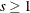, all data points are used.

Suppose q denotes the number of points in the local neighborhoods and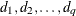denote the distances in increasing order of the q points closest to. The point at distance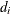fromis given a weight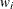in the local regression that decreases as the distance fromincreases. PROC LOESS uses a tricube weight function to define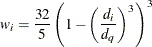If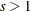, then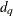is replaced by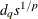in the previous formula, where p is the number of predictors in the model.

Finally, note that if a weight variable has been specified using a WEIGHT statement, thenis multiplied by the corresponding value of the specified weight variable.